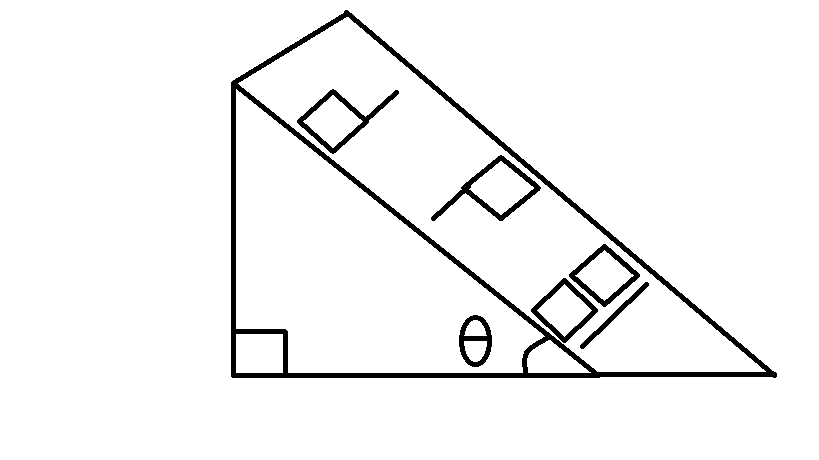# Mechanics Underkill

Two blocks $A$ and $B$ of equal masses are sliding down along parallel lines on a plane inclined at an angle of $\theta =45^\circ$ with the horizontal, as shown in the figure. The coefficients of kinetic friction are $0.2$ and $0.3$ for $A$ and $B,$ respectively. At $t=0,$ both the blocks are at rest, and block $A$ is $2$ meters behind block $B$.

Find the time (in seconds) and distance (in meters) from the initial position of $A$ to where the front faces of the blocks come in line on the inclined plane, as illustrated in the figure. $\big($Use $g=\SI[per-mode=symbol]{10}{\meter\per\second\squared}.\big)$

Input your answer as the sum of the answers for the time and distance, to 3 decimals.Source : JEE 2004

×